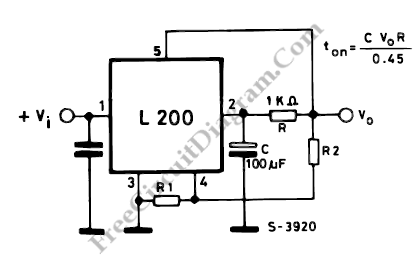# Soft Start Mechanism for L200 Voltage Regulator

The configuration in the circuit diagram below can be used when a slow rise time of the output voltage is required. We can find the rise time with following formula:

ton= CVoR
0.45Capacitor C is discharged when the switch position is ON and this will maintain the voltage at pin 2 low. The Vo follows the voltage at pin 2 at less than 0.45 V since a voltage of more than 0.45 V cannot be produced between pins 5 and pins 2.

Constant current ic is charge capacitor C, where

ic= Vsc/R

After the time ton, the output reaches it’s nominal value

Vo-Vsc = (Ic.ton)/C

ton=C.[(Vo-0.45)/0.45].R = CVoR/0.45

[Source: STMicroelectronics Application Note]